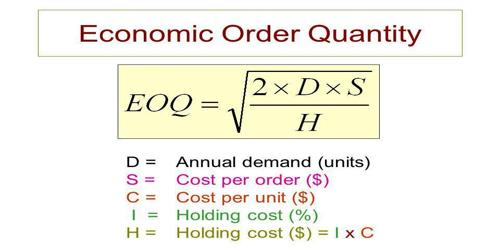# Assumptions are inherent in the Economic Order Quantity (EOQ) modelAssumptions are inherent in the Economic Order Quantity (EOQ) model

Economic order quantity (EOQ) is the order quantity of inventory that minimizes the total cost of inventory management. It is a measurement used in the field of Operations, Logistics, and Supply Management. By using this model, the companies can minimize the costs associated with the ordering and inventory holding.

The underlying assumption of the EOQ model – Following are the underlying assumptions for the EOQ model. Without these assumptions, the EOQ model cannot work to its optimal potential.

(a) The cost of the ordering remains constant.

(b) The demand rate for the year is known and evenly spread throughout the year.

(c) The lead time is not fluctuating (lead time is the latency time it takes a process to initiate and complete).

(d) No cash or settlement discounts are available, and the purchase price is constant for every item.

(e) The optimal plan is calculated for only one product.

(f) There is no delay in the replenishment of the stock, and the order is delivered in the quantity that was demanded, i.e. in the whole batch.

(g) That replenishment is made instantaneously, ie the whole batch is delivered at once.

(h) That costs to be used in EOQ calculations must be marginal costs. Fixed costs are excluded.

These underlying assumptions are the key to the economic order quantity model, and these assumptions help the companies to understand the shortcomings they are incurring in the application of this model.

Limitations of the economic order quantity model: It is necessary for the application of EOQ order that the demands remain constant throughout the year. It is also necessary that the inventory is delivered in full when the inventory levels reach zero.